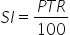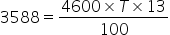Maths-
General
Easy

Question

# The simple interest on a fixed deposit of Rs. 4600 at the rate of 13% p.a. is Rs.3588. Find the term of the deposit.Hint:

## The correct answer is: T=6 years.

### Complete step by step solution:We calculate simple interest by the formula,…(i)where P is Principal amount, T is number of years and R is rate of interestHere, we have R = 13% ,SI = 3588,P = 4600 and T = ?On substituting the known values in (i), we getHence the term of the deposit, T = 6 years.#### With Turito Foundation.#### Get an Expert Advice From Turito.